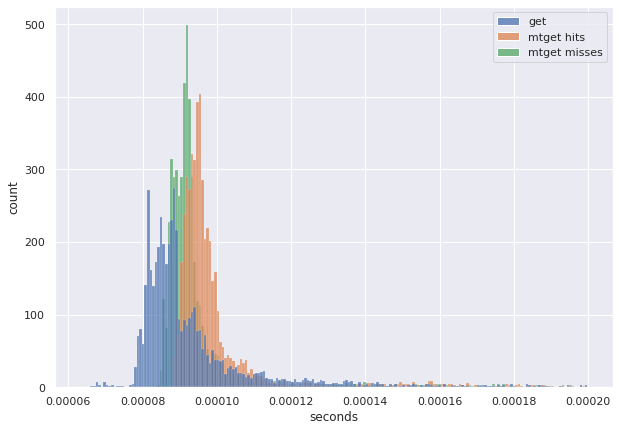The if modified since type of pattern for retrieving data from cache can save significant network bandwidth and compute cycles.

The data modification time reference should be from the client side and be sent as part of the request and not assumed on server side based on a non acknowledgement type of method like simply last time the client connected to the server.

This kind of pattern is possible on redis server side leveraging lua on redis, with very less overhead in terms of latency.

We can store the last modification time of a key in a hash and retrieve the value only if it is newer than the time client sent.

The following Lua snippets store/retrieve based on the modification time of key in a hash called `MY_KEYSTIME`.

Get based on modification time

``````local keys_mtime_hset = "MY_KEYSMTIME"
local key = KEYS
local mtime = tonumber(ARGV)

local key_mtime = redis.call('HGET', keys_mtime_hset, key)
key_mtime = tonumber(key_mtime)
-- if missing key in the hash set return the value of the key.
-- or key mtime > mtime
if not key_mtime or key_mtime > mtime then
return redis.call('GET', key)
end

return nil
``````

Set and update modification time

``````local keys_mtime_hset = "MY_KEYSMTIME"
local key = KEYS
local value = ARGV
local mtime = tonumber(ARGV)

redis.call('SET', key, value)
redis.call('HSET', keys_mtime_hset, key, mtime)
``````

Client sends the modification time while retrieving and setting the key. Redis Lua scripts are atomic.

The following python code illustrates a full working example.

``````mtime_get="""
local keys_mtime_hset = "MY_KEYSMTIME"
local key = KEYS
local mtime = tonumber(ARGV)

local key_mtime = redis.call('HGET', keys_mtime_hset, key)
key_mtime = tonumber(key_mtime)
-- if missing key in the hash set return the value of the key.
-- or key mtime > mtime
if not key_mtime or key_mtime > mtime then
return redis.call('GET', key)
end

return nil
"""

mtime_set="""
local keys_mtime_hset = "MY_KEYSMTIME"
local key = KEYS
local value = ARGV
local mtime = tonumber(ARGV)

redis.call('SET', key, value)
redis.call('HSET', keys_mtime_hset, key, mtime)
"""

m1 = int(time.time())
r = redis.Redis(host='localhost', port=6379)
MTGET=r.register_script(mtime_get)
MTSET=r.register_script(mtime_set)

t_k = "Hello"
t_v = "World"

r.delete(t_k)

print(MTGET(keys=[t_k], args=[m1]))

print(MTSET(keys=[t_k], args=[t_v, m1]))

print(r.get(t_k))

print(MTGET(keys=[t_k], args=[m1 - 100]))

print(MTGET(keys=[t_k], args=[m1 + 100]))
``````

Running it

``````None
None
b'World'
b'World'
None
``````

How much overhead will this incur ? A simple benchmark shows not a lot, since HGET is an O(1) operation.

``````REPEAT=10000
NUMBER=3

g = timeit.Timer("r.get(t_k)",globals=globals())
timings_get = g.repeat(repeat=REPEAT, number=NUMBER)

g = timeit.Timer("MTGET(keys=[t_k], args=[m1 - 100])",globals=globals())
timings_hits_mtget = g.repeat(repeat=REPEAT, number=NUMBER)

g = timeit.Timer("MTGET(keys=[t_k], args=[m1 + 100])",globals=globals())
timings_miss_mtget = g.repeat(repeat=REPEAT, number=NUMBER)
``````

Running and plotting the timings from this benchmark, x axis is time taken for calls.As expected there is a slight overhead, with plain get being the fastest and modification time based get the slowest. See this jupyter notebook for full working example and chart source.